## Recommended Posts

Hi

Has anyone reading this ever tried to create a 3D city using data from http://www.openstreetmap.org

What are the steps are involved?

Can you recommend any tutorials?

##### Share on other sites

There are free tutorial and working implementation somewhere in SESI's GitHub exist. Also, someone posted OSM importer 1-2 years ago here on odforum.

Here is a bit of my experience on this. At first, you should make yourself familiar with OSM format: nice wiki page on OSM specs . Export some small excerpt around your house and inspect it.

Simple Python OSM loader for xml rectangles exported from the website or from Overpass API:

```from collections import namedtuple
from xml.etree.cElementTree import parse

Bounds = namedtuple('Bounds', 'bounds')
Node = namedtuple('Node', 'id tags latlon')
Way = namedtuple('Way', 'id tags nodes')
Relation = namedtuple('Relation', 'id tags members')
Member = namedtuple('Member', 'ref role')

def _id(e):
try:
return long(e.attrib['id'])
except KeyError:
return long(e.attrib['ref'])

def _tags(e):
tags = {}
for tag in e.findall('tag'):
a = tag.attrib
tags[unicode(a['k'])] = unicode(a['v'])
return tags

root = parse(file_or_fp).getroot()
data = []

attrib = root.find('bounds').attrib
coords = map(float, (attrib['minlat'], attrib['minlon'],
attrib['maxlat'], attrib['maxlon']))
data.append(Bounds(tuple(coords)))

node_ids = {}
for e in root.findall('node'):
latlon = (float(e.attrib['lat']),
float(e.attrib['lon']))
node = Node(_id(e), _tags(e), latlon)
node_ids[node.id] = node
data.append(node)

way_ids = {}
for e in root.findall('way'):
nodes = [node_ids[_id(n)] for n in e.findall('nd')]
way = Way(_id(e), _tags(e), nodes)
way_ids[way.id] = way
data.append(way)

for e in root.findall('relation'):
members = []
for m in e.findall('member'):
ids = node_ids if m.attrib['type'] == 'node' else way_ids
members.append(Member(ids.get(_id(m)), m.attrib['role']))
data.append(Relation(_id(e), _tags(e), members))

return data```

It will convert OSM structure into Python objects. OSM file consists of Bounds, Nodes, Ways, Relations and its Members with their own simple attribute structures and optional child tags. "Useless" attributes were dropped, e.g. fully-described Nodes could look like this:

`namedtuple('Node', 'id visible version changeset timestamp user uid tags latlon')`

So, the above code can be modified to include any of those members too.

Nodes are similar to Houdini points with attributes. Isolated Nodes are used for information, rather than defining map features. Other nodes are just like primitives' points, they have no information and can be shared between primitives.

```import math

def mercator(lat, lon):
y = 180.0 / math.pi * math.log(math.tan(math.pi/4.0 + lat * (math.pi/180.0)/2.0)) * (x/lon)
return x, y

# Make nodes as points.
points = {}
for node in (n for n in data if type(n) is Node):
pt = geo.createPoint()
points[node.id] = pt

# Place to copy useful attributes defined on Node object, like:
# pt.setAttribValue('attrib', node.attrib)

x, y = mercator(*node.latlon)
pt.setPosition((x-pivotx, y-pivoty, 0.0))```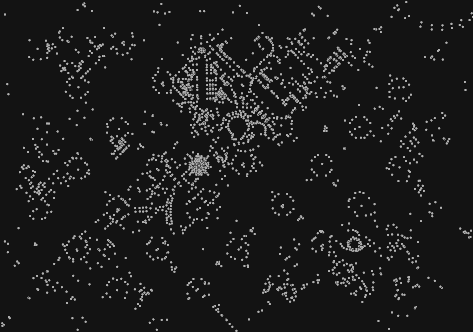Ways are like Houdini polygon curves. They have their attributes and refer to a bunch of Nodes:

```# Make ways as primitives.
for way in (w for w in data if type(w) is Way):
prim = geo.createPolygon()

# Place to copy useful attributes defined on Way object, like:
# prim.setAttribValue('attrib', way.attrib)

prim.setIsClosed(way.nodes is way.nodes[-1])
if prim.isClosed():
way.nodes.pop()

pts = [points[node.id] for node in way.nodes]

# Gauss's area determines the winding order.
gauss_area = 0.0
for i in range(len(pts)):
ax, ay, _ = pts[i].position()
bx, by, _ = pts[(i+1) % len(pts)].position()
gauss_area += (bx-ax) * (by+ay)
if gauss_area < 0.0:
pts.reverse()

for pt in pts:
```

That should define most of the city: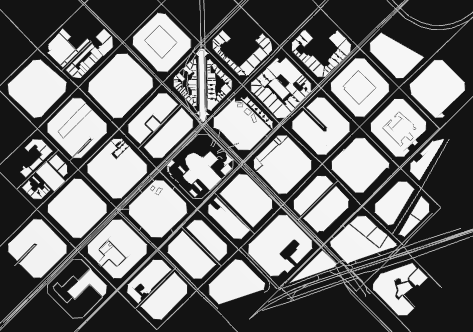The trickiest part is to handle Relations and Members. This is important part of OSM specs defining areas and complex buildings sharing some common relation: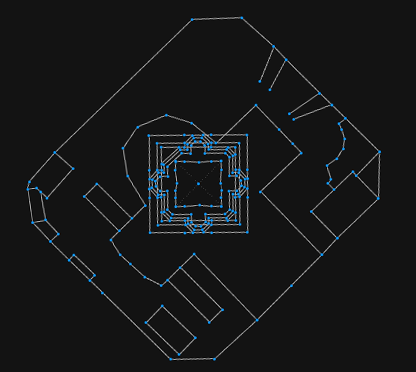Basically, they help to define what is a fence, a building block or inner open space. They make use of tags, which is totally human-generated content. There are many challenges with them if you try to make them generic enough to handle any input map.

Even without proper Relations handling, when we made primitives from Ways we already had something decent, that can work on large-scale: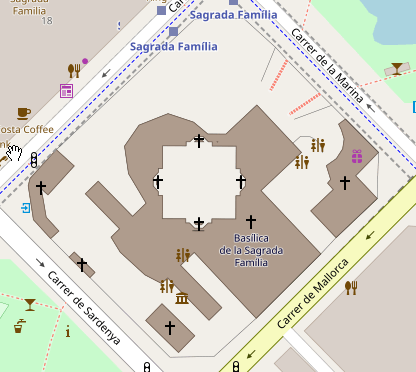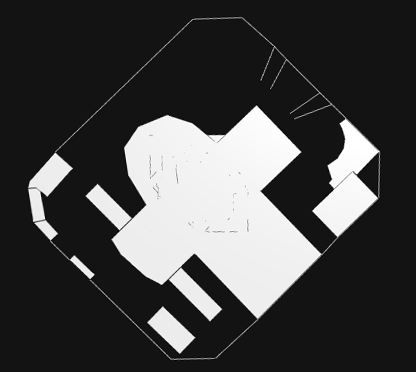Currently, this is just a stalled project on my disk, I have no time on it at this moment, so, I'll simply upload it there:

Edited by f1480187
•3

##### Share on other sites

Is it possible to derive the driving direction from the imported OSM data? It can be seen as arrows on oneway streets on openstreetmap.org and my mapping friends tell me this is stored in the Node in OSM but I can't figure out if that information is available in in Houdini using the OSM_Import node.

## Create an account

Register a new account